Pi has a very intrensic value in the feild of mathematics and science.The number π(Pi) has many remarkable properties—among other things, it is irrational and indeed transcendental—and its presence in mathematical formulas is widespread.

But ,what if Pi is wrong?                                                                                           We have been using pi for so long time that now we feel insecure if someone questions pi.

Some communities  have been talking on how replacing pi with tau, which will be helpful for basic studies and general understanding . This communities proudly call themselves Tauist and celebrate Tau Day on 28th June since Tau is two times Pi which means Tau=6.28……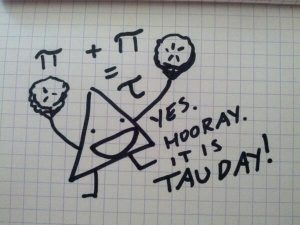The basic concept behind Pi when it was discovered during the Babylonian times  was that it is the ratio  of Circumference of circle to Diameter of Circle. During that time it was hard to find out radius of the circle so they used diameter to find the ratio constant Pi . But when we look at circumference of circle it is 2π times radius and why not just π times radius . The basic question all Tauist ask is why such a constant is being used which needs a more constant value to complete .

On dividing a Circle into 1/4th, the angle substituted at centre in terms of radian is Pi/2, but it is 1/4th of a circle and if we replace pi by tau the angle substituted at the centre will be Tau /4. It just became simpler.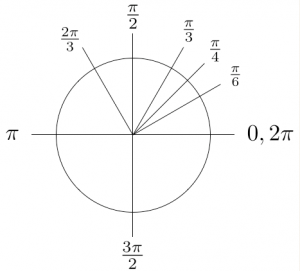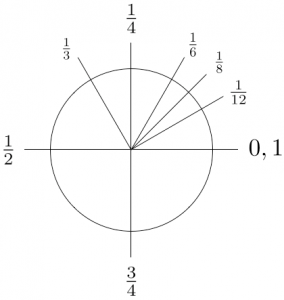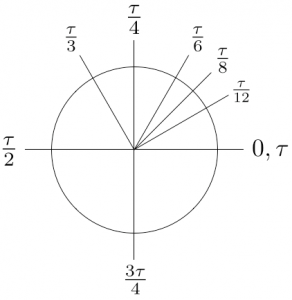Everywhere in mathematics, we see use of 2π in most of formulae around. But not just in mathematics , even in classical physics the formulae have 2π as most basic constant for e.g The time period of pendulum is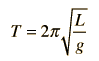where T is time period  , but what if we change pi to tau the formula would just become  more  simpler to learn .

We see use of 2π everywhere in mathematics and physics ,and since mathematics is known for its elegance ,So people are arguing to change it to Tau which will just make understanding and learning  a bit more simpler .But it will be very hard to remove a so well established Pi which can cause global confusion.It depends on you to choose which side ,but since it is Tau day-

Happy Tau Day!